• 平面向量内积的坐标表示.ppt
2020-12-18 23:08:43

7.10 平面向量内积的坐标表示 1、掌握用直角坐标计算向量的内积公式。 2、掌握向量长度、垂直的坐标表示及夹角公式，掌握平面两点间距离公式； 学习 目标 重点 难点 课型 学法 通过推导和题组训练，理解并掌握向量长度、垂直、夹角及距离公式。 能准确运用向量内积的坐标表示长度、垂直、夹角及距离公式等结论，解决有关问题。 新 课 启发式、练习法 达标过程 一、复习导入 1. (5,7) 我们学过两向量的和与差可以转化为它们相应的坐标来运算,那么怎样用 ？？？ 二、新课学习 1、平面向量内积的坐标表示 如图， 是x轴上的单位向量， 是y轴上的单位向量， x y o B(b1,b2) A(a1,a2) 1 1 0 . . ； 下面研究怎样用 设两个非零向量 的坐标是(a1,a2), 的坐标是(b1,b2), 则 o x B(b1,b2) A(a1,a2) y 那么 x o (b1,b2) (a1,a2) y 根据平面向量内积的坐标表示，向量的内积的运算可转化为向量的坐标运算。 故两个向量的内积等于它们横坐标的乘积与纵坐标乘积之和。 热身 解： 探究新知 2、向量的长度和两点间的距离公式 3、两向量垂直 4、两向量夹角公式的坐标运算 收获到了 三、基本技能的形成与巩固 解： -15 5 不垂直 垂直 1.填空 抢答题 例2 已知A(1，2)，B(2，3)，C(-2，5)，证明?ABC是直角三角形. A(1,2) B(2,3) C(-2,5) x 0 y 注：两个向量的内积是否为零是判断相应的两条直线是否垂直的重要方法之一。 如证明四边形是矩形，三角形的高，菱形对角线垂直等。 已知?ABC三个顶点坐标A( -1，2)， B(3，1)，C(2，-3)， 求证：?ABC是等腰直角三角形. 小结 (1)掌握平面向量内积的坐标表示，即两个向量的内积等于它们对应坐标的乘积之和； (2)要学会运用平面向量内积的坐标表示解决有关长度、角度及垂直问题. 节清内容 　　课本Ｐ36 　　　 Ａ组　1、2、3、5、7中任选一题, 4. 　　 　　　学习 要有竹子样的坚韧的品质

更多相关内容
• 通过这几天对平面向量的研究，我是越来越有兴趣了，因为平面向量让我开拓眼界，给我一个新的认知角度来看待世界，“问题是最好的老师”，我讲通过自己提出问题，来进行解决总结，让自己对平面认识深刻更好利用。...

通过这几天对平面向量的研究，我是越来越有兴趣了，因为平面向量让我开拓眼界，给我一个新的认知角度来看待世界，“问题是最好的老师”，我讲通过自己提出问题，来进行解决和总结，让自己对平面认识深刻和更好利用。

一、抽象能力：

抽象是从众多的事物中抽取出共同的、本质性的特征而舍弃其非本质的特征.例如苹果、香蕉、生梨、葡萄、桃子等,它们共同的特性就是水果.得出水果概念的过程,就是一个抽象的过程。平面的抽象是把以前平面只有大小没有方向的数量，把大小和方向不相关的两个概念进行抽象，抽象成在二维平面内既有方向又有大小的量这就是平面向量。向量是如何相等的？

长度相等且方向相同的两个向量叫做相等的向量。即：若向量a与向量b相等，则记作向量a=向量b，相等向量互相平行，任意两个相等的非零向量都可以用同一有向线段来表示，并且与有向线段的起点无关。把没有长度的0，抽象成了零向量，零向量的方向与任一向量平行，垂直。零向量可以有很多方向，却只有一个长度，我们可以把大家公认的长度，抽象为单位长度，我们在平面向量里抽象为单位向量。单位向量是指模等于1的向量。由于是非零向量，单位向量具有确定的方向。单位向量有无数个。一个非零向量除以它的模，可得所需单位向量。一个单位向量的平面直角坐标系上的坐标表示可以是：(n,k) ，则有n²+k²=1。

二、高维思考

例如：大家都知道什么是“画地为牢”。就是你在一张纸上画一只老虎，然后你想困住它，怎么弄？哈哈，画地为牢，你只要在纸上画上一个牢，把纸里的老虎困住就行了。为什么老虎走不出来？因为在一张纸的二维平面里面，没有“高”这个概念。正因为如此，老虎才被人画牢所困。那么三维的老虎呢？三维的老虎需要真正的笼子，因为二维的牢困住二维的虎，三维的虎当然要用三维的牢才可以困住。也就可以说，二维的牢困不住三维的虎，因为三维的虎比二维的虎多出一个“高”来。就是说，在维度上，我们可以认为三维比二维高级。

在平面向量方面我们就可以把方向与长度做为整体来进行考虑，把两个不想关的概念“方向”和“长度”抽象成一个整体为平面向量。对方向和长度我们可以利用平面向量的维度来考虑，如果没有没有学习过平面向量，我们就没有这个维度来认知长度和方向的统一。我们在生活中整体考虑有好多，方向：我们常见的方向有上北下南，左西又动，北偏西多少度。南偏西多少度等等可以利用这些来表示方向，但是我们可以把这些表示都可以看成一个整体，什么是不变的，在这里“方向就是不变的”所以我们可以把方向看做成一个整体。面积：正方形的面积，三角形的面积，圆形的面积，我们都不用考虑他的公式，我们可以把他们做成一个整体来看，他们的面积就是一个整体。数字也可以作为一个整体，不管你是无理数还是有理数，他都是一个数，一个数除以一个数都会得到一个数。速度：只要你动了一定会出现一个距离和时间，这样我们就得到了一个速度。不管你是走的，还是坐车，还是坐飞机他们都是速度。

我大胆的猜测一下只要是比的话，我们都可以看成是整体，比出来的东西有一个是不变的。

三、平面向量三角形、四边形法则

什么是平面向量三角形法则：AB+BC=AC，这种计算法则叫做向量加法的三角形法则，首尾相连、连接首尾、指向终点。

平面向量三角形和平面四边形法则是如何转换的：它是一种共点力的合成法则．这一法则通以表示两个共点力的有向线段为邻边作一平行四边形，该两邻边之间的对角线即表示这两个力的合力的大小和方向．有时为了方便也可以只画出一半的平行四边形,也就是力的三角形法则.即把两个共点力中的一个平移,使它们首尾相接,再用一条线与两个力连接成一个三角形,第三边就是合力.  三角形定则平行四边形定则的实质是一样的。

三角形法则和平行四边形法则的思想含义：把数学和物理等学科统一起来了，如可以利用具有几何意义的复数运算来定义向量的运算，把坐标平面上的点用向量表示出来，并把向量的几何表示用于研究几何与三角问题，利用三角形和平面四边形法则多了一种角度，看问题更简单了，把复杂的问题变成简单的问题，更容易解决。

四、多向量为什么可以只分解为两个正交分量：

其实把平面向量进行正交分解的目的就是为了进行坐标运算。设i，j表示平面直角坐标系里的标准正交基（满足i⊥j，并且|i|=|j|=1，也就是说i，j是单位正交向量组）。这样任意一个向量a可以分解为如下形式：a=xi+yj，其中(x,y)就是向量a在以i，j为基的平面内的坐标。显然x和y就是a在i，j方向上的投影，因此x=|a|cos<a,i>,y=|a|cos<a,j>。在进行向量运算的时候可以省去基向量，这样向量的运算也就转化成了坐标的运算。这也可以看出，选取的基不同，a分解后的结果也不同，因此平面向量的正交分解不是唯一的。再深入研究标准正交基的性质，若是xi+yj=0，则一定可以得到x=y=0，也就是说i和j是线性无关的。因此对于任意一组线性无关的向量α和β均可以进行运算使其变为单位正交向量。这个过程就是施密特正交化。施密特正交化不仅可以在平面向量中应用，还可以在n为线性空间中应用。

正交分解带来的启迪：1.力是矢量F′在X轴Y轴上的分矢量F′x和F′y是矢量，分量为正值表示分矢量的方向跟坐标轴的方向相同，分量为负值表示分矢量的方向跟坐标轴的方向相反。2．确定矢量正交分量的坐标轴，不一定是取竖直方向和水平方向。例如，分析物体在斜面上的受力情况，一般选取x轴与斜面平行，y轴与斜面垂直。坐标轴的选取是以使问题的分析简化为原则。通常选取坐标轴的方法是：选取一条坐标轴与物体运动的加速度的方向相同（包括处理物体在斜面上运动的问题），以求使物体沿另一条坐标轴的加速度为零，这样就可得到外力在该坐标轴上的分量之和为零，从而给解题带来方便。3、正交分解是先将线性方程组Ax=b的系数矩阵A分解为一个正交矩阵Q和一个对角可逆上三角矩阵R的乘积。然后通过求解上三角方程组Rx=Qb而求得原方程组的解，这种方法一般比三角分解法运算量大，但数值稳定性较好。

五、有了向量的认知的能力，思考是如何飞翔的

1、我们在一开始当学习向量的时候，我们一定接触到了很多新的知识点，如果在没有互联网下，在老师讲课下，我们只能学习向量的本身知识，而不能学习到向量带来的思想，我们首先对平面向量有一个基本认识，在学习平面向量过程中，我们不仅要注重平面向量的结论，我们更要注重学习平面向量过程，我们要在向量里面学习的思想挺多，我们要把这些思想应用起来。

2、平面向量是解决数学问题的一种基本工具，在运用向量法处理函数问题、三角问题，函数问题时，给传统的解法注入了新的活力，为数学中的推理、运算开辟了新的途径，实现了几何的代数化，为数和形的结合做了很好铺垫，而且运用向量法解题在知识的联系、转化和问题的解决过程中有着其他知识点难以启迪的优势，由于向量这一新的视角，进一步扩宽了思维的渠道，要从思想方法上研究平面向量的实质，修复原有的认知。

3、平面向量与其它知识的联系较多，特别是三角、解析几何、立体几何、复数等。平面向量既有代数形式又有几何意义，对于一道平面向量的问题，我们不仅仅可以利用代数方法又可以从几何方法的角度考虑。

4、平面向量中有着丰富的数学思想方法，在解决有关向量问题时，我要是能统分运用这些数学思想方法，可使许多问题获得简洁、巧妙解决。1、方程思想2、树形结合思想就是将抽象数学语言与直观、具体的图形结合起来，通过数与形的相互转化，达到化难为易，化繁为简的目的。3、分类讨论就是由于某种原因使得所给对象不能进行统一研究时，对研究对象进行分类讨论，然后对每一类结果分别研究，得出每一类结论，分类讨论实质上是一种“化整为零，各个击破”。

5、平面向量给我一个新的角度去认知世界，让我去开阔眼界，让我提升了一个维度，不在局限，有了平面向量的维度我可以发现更多有趣的事，更可以去创造。

展开全文• ## 平面向量

热门讨论 2020-11-15 20:37:01
经过一周的平面向量的学习，让我对平面向量有了新的认识。 向量的出现可以方便我们的计算三角函数，开创了研究几何的问题的新方法。通过演绎推理 ，建立几何 关系，因此，他给出的几何论证严禁且优雅，给人极大的...

经过一周的平面向量的学习，让我对平面向量有了新的认识。
向量的出现可以方便我们的计算三角函数，开创了研究几何的问题的新方法。通过演绎推理 ，建立几何 关系，因此，他给出的几何论证严禁且优雅，给人极大的享受。
向量的定义：既有大小，又有方向的量，在物理学称为矢量。
单位向量：长度等于一个单位的向量。
测量一般是先确定单位，后测量长度，而在单位向量这里却可以先测量长度，回头再去看单位，它打破了普通计算的方法，颠倒了测量中的顺序，并且单位可大可小，只是一旦确定就不能再改变，在固定条件下单位向量记做为1。
零向量：长度等于0的向量。
长度为0，却有方向，只是方向不定。
方向和长度相等的向量，为什么是相等的
向量的定义时既有大小又有方向的量，当方向相同时，这两个向量是共线向量，因为向量只要长度和方向不变是可以任意移动的，因此他们组成了一个维度的有朝向的一条线段，长度为较长的那条向量的长度.
在这个问题上，首先要清楚向量的定义，否则就无法为结论提供充足的证据。在解决其他问题上同样要本着严谨的态度提供可靠的依据，结论缺少依据不止大多数人不会认可，同样对后面向量的更深一步的计算也会站不住脚跟，变成无从下手的状态。这里提两个概念，一是公理；二是中间定理。
公理：在数学中，公理这一词被用于两种相关但相异的意思之下——逻辑公理和非逻辑公理。在这两种意义之下，公理都是用来推导其他命题的起点。和定理不同，一个公理（除非有冗余的）不能被其他公理推导出来，否则它就不是起点本身，而是能够从起点得出的某种结果—可以干脆被归为定理了。中间定理：也叫定理，是经过受逻辑限制的证明为真的陈述。一般来说，在数学中，只有重要或有趣的陈述才叫定理。证明定理是数学的中心活动。
公理与定理也要区分定理：已证实。对于全部情形都得到了证明公理：未证伪。无法穷举全部情形，但尚未发现反例
学习中高维思考
在向量的学习中，我们认识到运用向量要考虑两个方面：长度和方向。也就是说要整体性考虑问题，不能丢失条件，向量不考虑方向就和线段没有区别，就像物体表面积不考虑长或宽就无法得出结果一样，就像血液一样遍布全身各处。
在我们学习过程中，还有很多需要进行整体性的考虑，例如学习英语，我们学习英语单词时不仅要看单词拼写和单词意思，还要看的一点就是单词的音标。缺少音标指导准确的发音，在听、说、读、写上只剩下写能够做到了，其他三个听、说、读都需要音标参与才能做到，整体性思考的重要性就凸显出来了。所以在英语单词学习上音标、单词拼写、词义缺一不可。还有单词的来源，和单词的延伸意思。
这样的整体性思考遍布了学习的整个过程，我们站在巨人的肩膀上学习，享受着前人总结的成果，所以为了给后人也提供一个结实的肩膀，我们要吸收前人的经验，努力为后人搭建更牢固的桥梁。
平面向量的计算法则
平面向量中的两大法则
（1）三角形法则已知非零非共线向量a和b,在平面内任取一点A,作向量AB=向量a,过B点作向量BC=向量b,连接AC,得向量AC.
则向量AB+向量BC=向量AC.
即,向量a+向量b=向量AC.
∵三个向量构成的图形正好是一个三角形,
∴此法则叫做向量的三角形法则.
向量三角形法则的扩展:在平面内,有n个向量,首尾相连,最后一个向量的末端与第一个向量的始端相连,则最后这一个向量(方向由第一个向量的始端指向最末一个向量的末端)就是n个向量之和。
平面向量平行四边形法则∴向量AC=向量a+向量b。
向量加法的三角形法则和平行四边形法则揭示了：任意一个非零向量，都可以分解出任意两个方向的分量,只要这两个分量平移延长后组成的图形满足平行四边形。
任意一个非零向量可以有无数组分量组成，且不限制于固定一个平面，所以说任意一个非零向量都有多种变换和组成的可能，就像世间万物多种变化，看似不同，实则同源。
多向量为什么只可以分解成两个正交分解
将一个力分解为Fx和Fy两个相互垂直的分力的方法，叫作力的正交分解。从力的矢量性来看，是力F的分矢量；从力的计算来看，力的方向可以用正负号来表示，分量为正值表示分矢量的方向跟规定的正方向相同，分量为负值表示分矢量的方向跟规定的正方向相反。这样，就可以把力的矢量运算转变成代数运算．所以，力的正交分解法是处理力的合成分解问题的最重要的方法，是一种解析法．特别是多力作用于同一物体时。而在向量的角度看，只要大小和方向不变，向量是相等的，因此多向量只可以分解成两个正交分解。向量可以存在于一维空间、二维空间或三维空间，向量和标量不同跨越了多个空间维度。
启迪
1.抽象能力：将一个向量抽象成两个正交分量
2.高维思考能力：将多个方向，多个长度，整体化统一成两个方向并且成90°；抽象和高维思考于分解和合成十分相像，这两种能能力正是我们需要的。
向量的认知能力，思想是如何飞翔的
平面向量是解决数学问题的一种基本工具，在运用向量法处理函数问题、三角问题，函数问题时，给传统的解法注入了新的活力，为数学中的推理、运算开辟了新的途径，实现了几何的代数化，为数和形的结合做了很好铺垫，而且运用向量法解题在知识的联系、转化和问题的解决过程中有着其他知识点难以启迪的优势，由于向量这一新的视角，进一步扩宽了思维的渠道，要从思想方法上研究平面向量的实质，修复原有的认知。
向量的来源我们看作是由物理的重力方向得来，既有长短，同时有方向。物理学和我们的生活息息相关，向量在物理中的‘称呼’被叫做‘矢量’，常常看到的有矢量图、矢量文件等，其中矢量图包含独立的分离图像，可以自由无限制的重新组合，它的特点是放大后图像不会失真。相对于情况单一的标量，矢量代表更复杂和认知和理解，了解了向量的概念，就如同学会了降维打击，把一维的标量思想升级成三维的矢量思想。让我们看待事物越发全面和深刻。
平面向量与其它知识的联系较多，特别是三角、解析几何、立体几何、复数等。平面向量既有代数形式又有几何意义，对于一道平面向量的问题，我们不仅仅可以利用代数方法又可以从几何方法的角度考虑。
平面向量中有着丰富的数学思想方法，在解决有关向量问题时，我要是能统分运用这些数学思想方法，可使许多问题获得简洁、巧妙解决。1、方程思想2、树形结合思想就是将抽象数学语言与直观、具体的图形结合起来，通过数与形的相互转化，达到化难为易，化繁为简的目的。3、分类讨论就是由于某种原因使得所给对象不能进行统一研究时，对研究对象进行分类讨论，然后对每一类结果分别研究，得出每一类结论，分类讨论实质上是一种“化整为零，各个击破”。
向量的学习告诉我们自己不知道的领域有多深奥又浅显的知识，教会敬畏科学，看到自己的不足，在不同的领域运用大众所知的知识也是一种智慧。学科之间没有界限，但是我们的认知给我们的思想制造了障碍，在今后的学习，动用自己全部的所知所想，不妄下结论，随意给事物定性，站在巨人的肩膀上，用普世的公理，创造出新的定理。平面向量给我一个新的角度去认知世界，让我去开阔眼界，让我提升了一个维度，不在局限，有了平面向量的维度我可以发现更多有趣的事，更可以去创造。
小伙伴们让我们站在巨人的肩膀上。去创造，去发现。

展开全文• SVM是机器学习领域非常知名的模型。本文讲解SVM的最大间隔分类器、模型原理、核函数与核技巧等重要知识点，并附上线性核函数、多项式核函数高斯核函数的Python代码实践。作者：韩信子@ShowMeAI
教程地址http://www.showmeai.tech/tutorials/34
本文地址http://www.showmeai.tech/article-detail/196
声明：版权所有，转载请联系平台与作者并注明出处

# 引言

本篇我们要讲解的模型是大名鼎鼎的支持向量机SVM，这是曾经在机器学习界有着近乎「垄断」地位的模型，影响力持续了好多年。直至今日，即使深度学习神经网络的影响力逐渐增强，但SVM在中小型数据集上依旧有着可以和神经网络抗衡的极好效果和模型鲁棒性。

支持向量机学习方法，针对不同的情况，有由简至繁的不同模型：

• 线性可分支持向量机（linear support vector machine in linearly separable case）：训练数据线性可分的情况下，通过硬间隔最大化（hard margin maximization），学习一个线性的分类器，即线性可分支持向量机（亦称作硬间隔支持向量机）。

• 线性支持向量机（linear support vector machine）：训练数据近似线性可分的情况下，通过软间隔最大化（soft margin maximization），学习一个线性的分类器，称作线性支持向量机（又叫软间隔支持向量机）。

• 非线性支持向量机（non-linear support vector machine）：训练数据线性不可分的情况下，通过使用核技巧（kernel trick）及软间隔最大化，学习非线性分类器，称作非线性支持向量机。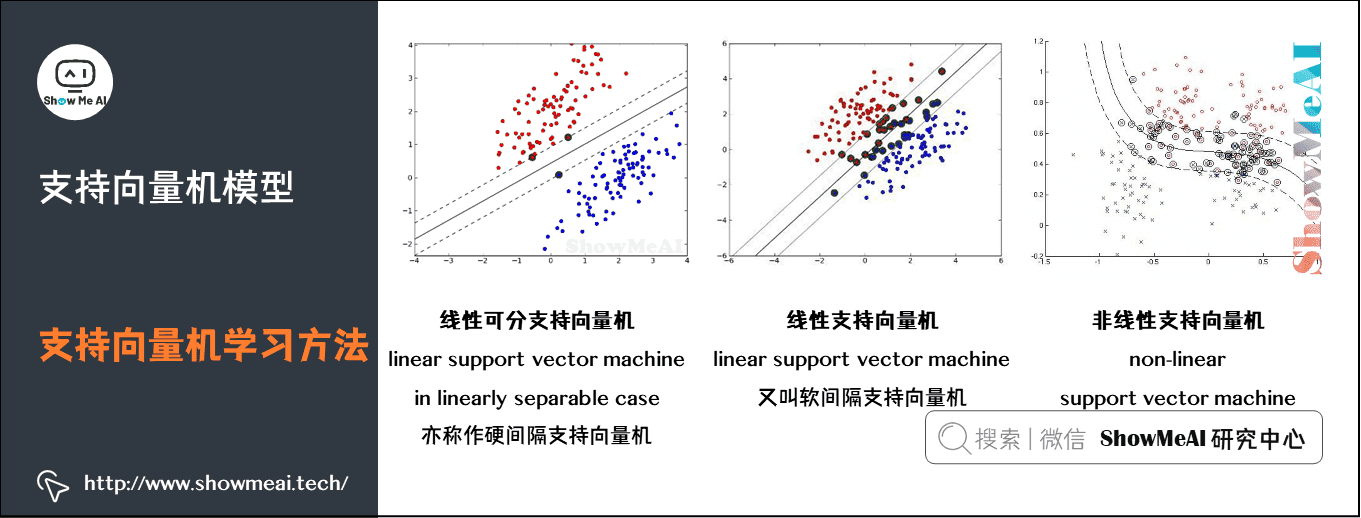支持向量机可以借助核技巧完成复杂场景下的非线性分类，当输入空间为欧式空间或离散集合、特征空间为希尔贝特空间时，核函数（kernel function）表示将输入从输入空间映射到特征空间得到的特征向量之间的内积。

通过使用核函数可以学习非线性支持向量机，等价于隐式地在高维的特征空间中学习线性支持向量机。这样的方法称为核技巧。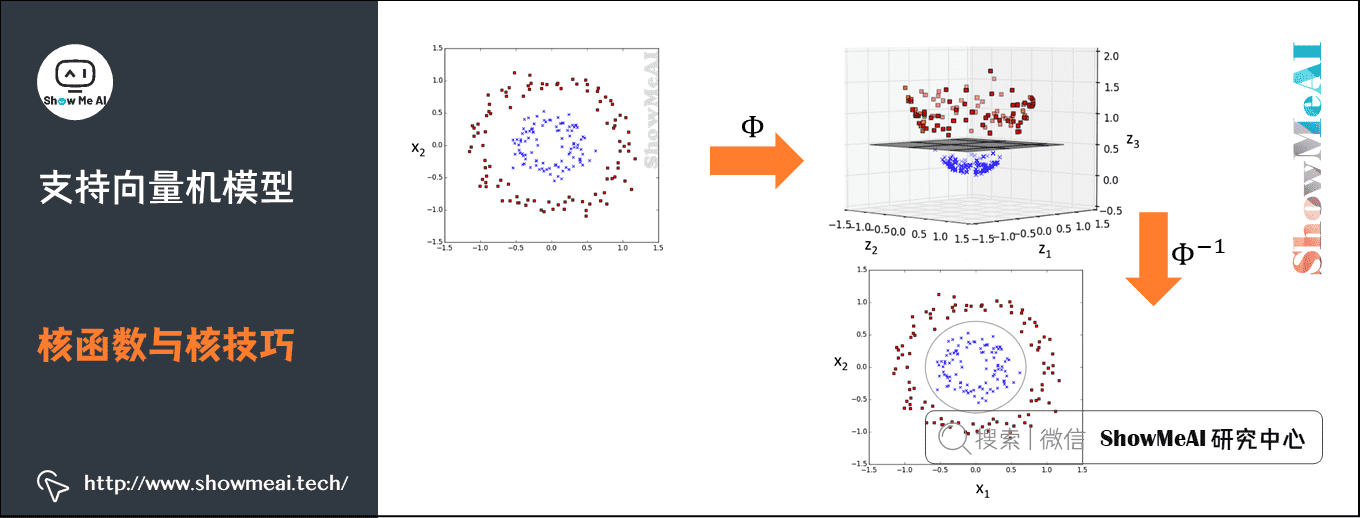# 1.最大间隔分类器

## 1）分类问题与线性模型

分类问题是监督学习的一个核心问题。在监督学习中，当输出变量取有限个离散值时，预测问题便成为分类问题。实际生活中，有很多问题的本质都是分类，如识别某种模式：文本分类、分词、词性标注、图像内容识别和目标检测等。分类问题的数学理解是空间划分（或者寻找不同类别的决策边界），如下图所示是一个简单的线性分类器（这部分更详细的讲解参考ShowMeAI文章 图解机器学习 | 机器学习基础知识图解机器学习 | 逻辑回归算法详解）。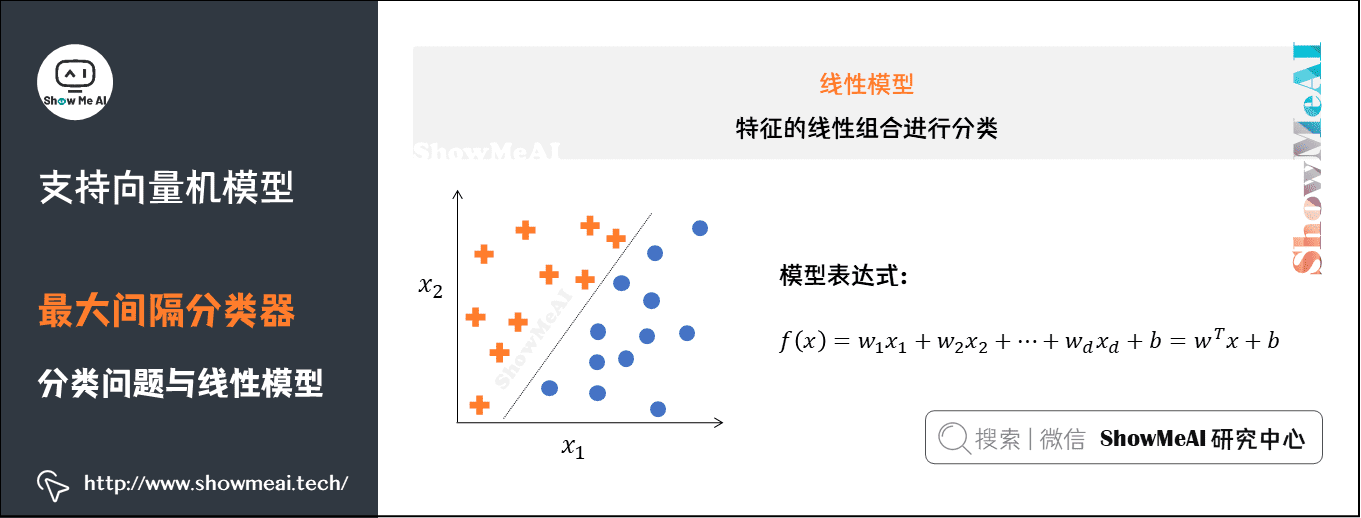## 2）最大间隔分类器

不同的模型在解决分类问题时，会有不同的处理方式，直观上看，我们会使用不同的决策边界对样本进行划分。

如下图中「冰墩墩」与「雪容融」两类样本点，我们对其进行分类，可以完成该分类任务的决策边界有无数个。而我们这里介绍到的SVM模型，要求更高一些，它不仅仅希望把两类样本点区分开，还希望找到鲁棒性最高、稳定性最好的决策边界（对应图中的黑色直线）。这个决策边界与两侧「最近」的数据点有着「最大」的距离，这意味着决策边界具有最强的容错性，不容易受到噪声数据的干扰。直观的理解就是，如果决策边界抖动，最不容易「撞上」样本点或者进而导致误判。

# 2.支持向量机详解

## 1）线性可分SVM与硬间隔最大化

我们要找到把下图中红蓝两色的图标点分开的最优分界线。令红色的图标点 = + 1 =+1 ，蓝色的图标的点 = − 1 =-1 ，直线 f ( x ) = w ⋅ x + b f(x) = \boldsymbol{w}\cdot \boldsymbol{x} + b ，这里 w 、 x w、x 是向量，其实公式等价于 f ( x ) = w 1 x 1 + w 2 x 2 + … + w n x n + b f(x) = w_1x_1 + w_2x_2 +…+ w_nx_n + b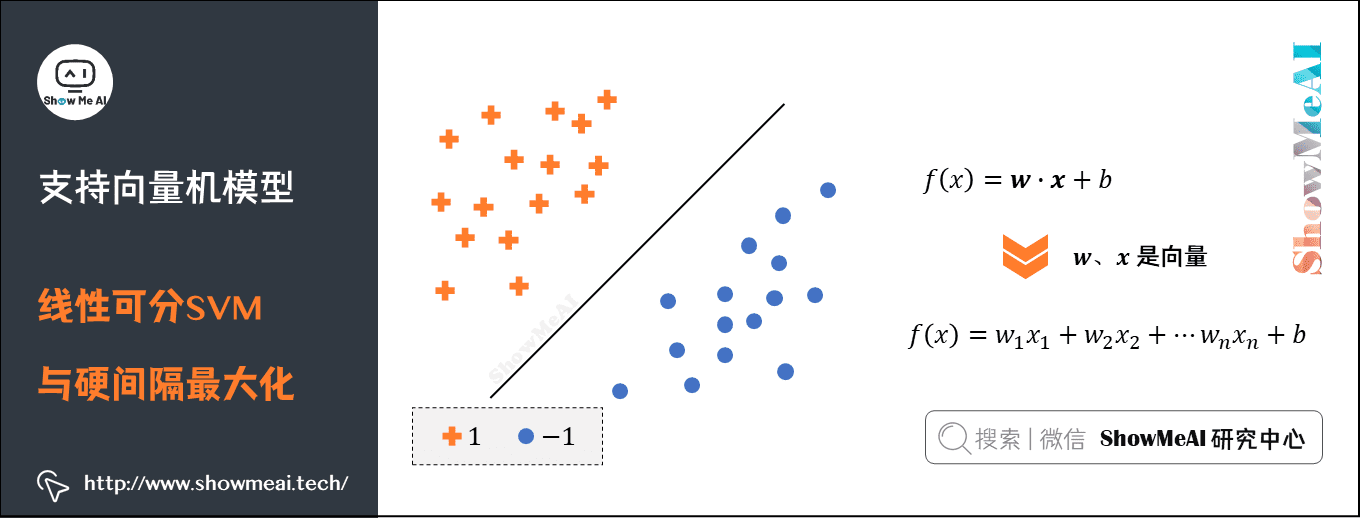• 当向量 x x 为二维向量时， f ( x ) f(x) 表示二维空间中的一条直线。

• 当向量 x x 为三维向量时， f ( x ) f(x) 表示三维空间中的一个平面。

• 当向量 x x n n 维向量（ f n > 3 fn>3 ）时， f ( x ) f(x) 表示 n n 维空间中的 n − 1 n-1 维超平面。

当有一个新的点 x x 需要预测属于哪个分类的时候，我们用 s g n ( f ( x ) ) sgn(f(x)) 就可以预测了。 s g n sgn 表示符号函数：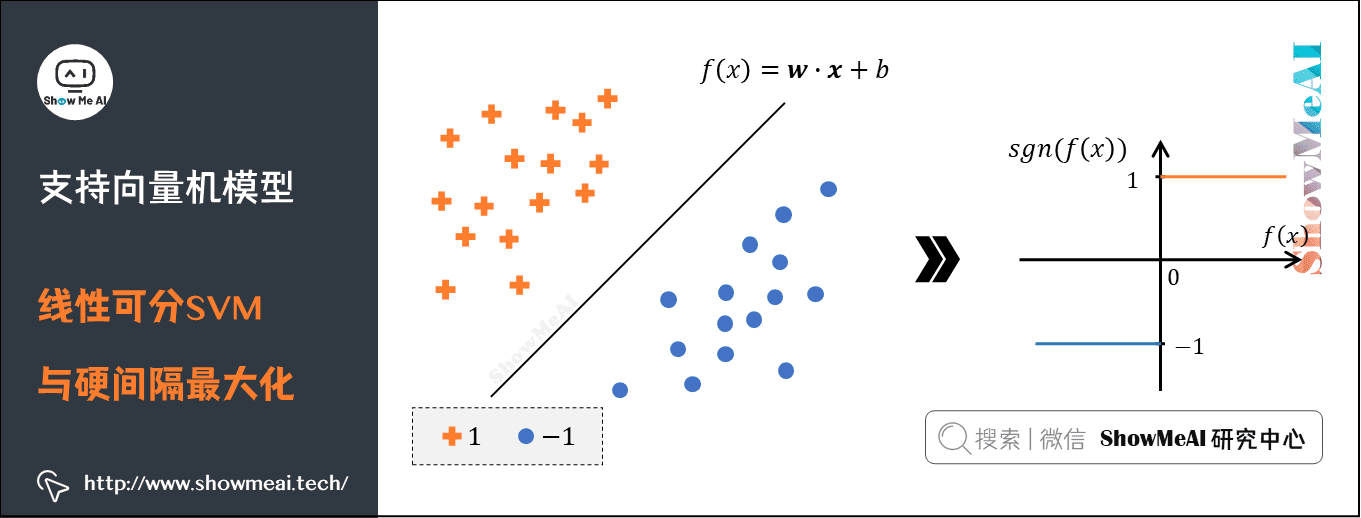• f ( x ) > 0 f(x)>0 的时候， s g n ( f ( x ) ) = + 1 sgn(f(x)) = +1

• f ( x ) < 0 f(x) < 0 的时候 s g n ( f ( x ) ) = – 1 sgn(f(x)) = –1

回到重点，我们怎样才能取得一个最优的划分直线 f ( x ) f(x) 呢？下图的直线表示几条可能的 f ( x ) f(x)我们希望这条直线满足「最大间隔」原则，也就是如下图的形态。图中位于红色和蓝色线上的图标就是所谓的支持向量（support vector）。决策边界就是 f ( x ) f(x) ，红色和蓝色的线是支持向量（support vector）所在的面，红色、蓝色线之间的间隙就是我们要最大化的分类间的间隙M（Margin Width）。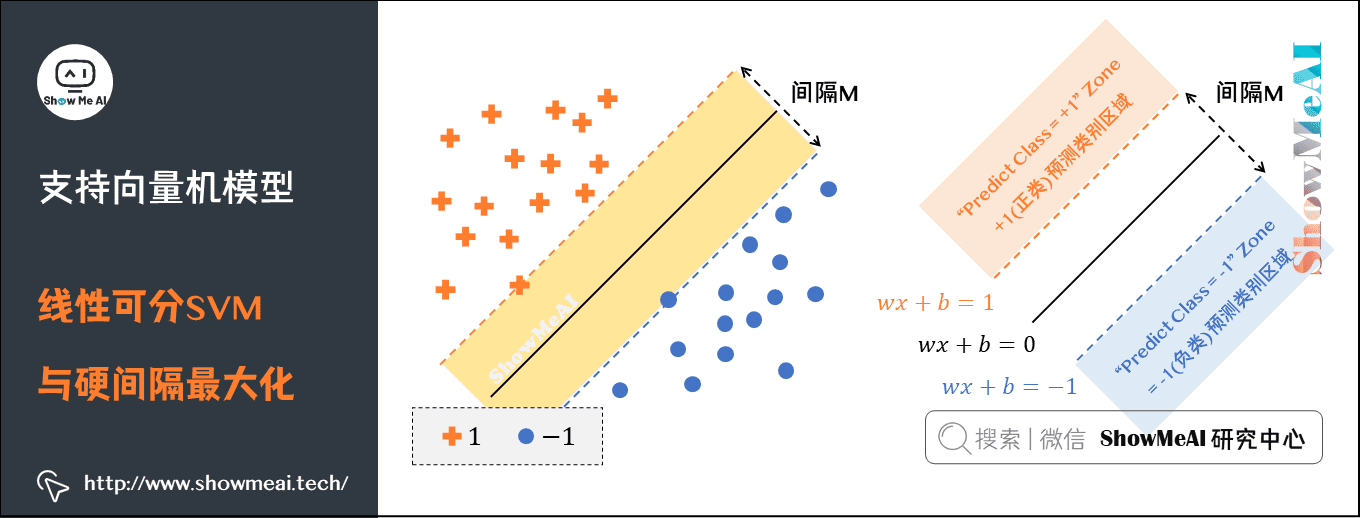这里直接给出间隔M的计算公式： M = 2 ∥ w ∥ M=\frac{2}{\left \| w \right \| }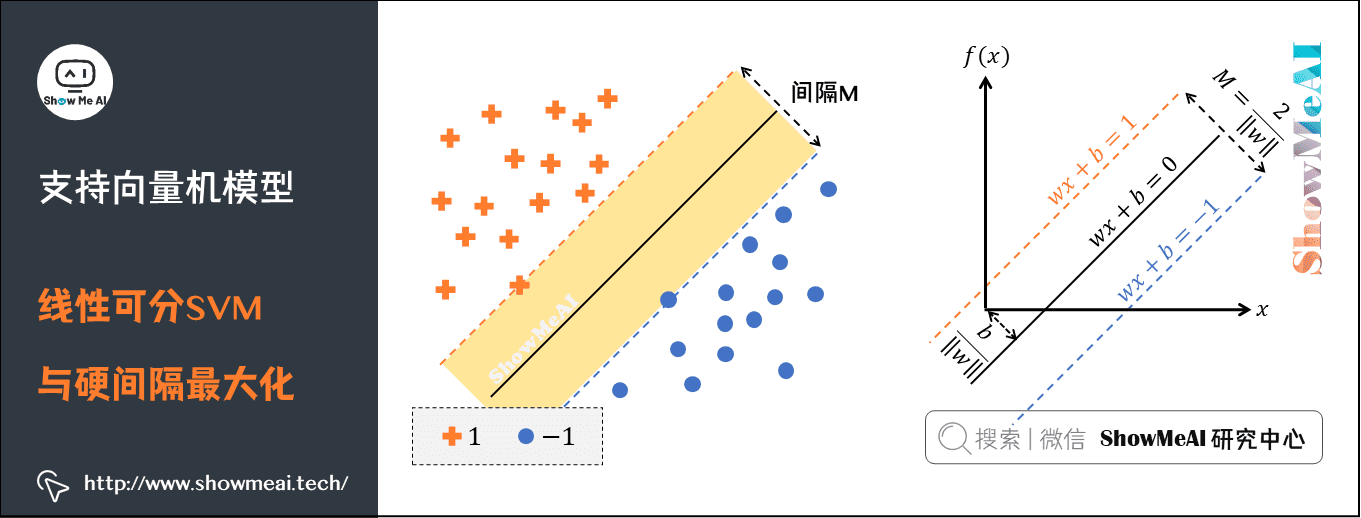SVM要求解能够正确划分训练数据集并且「几何间隔」最大的分离超平面。

如下图所示， w ⋅ x + b = 0 \boldsymbol{w} \cdot \boldsymbol{x}+b=0 即为分离超平面。对于线性可分的数据集来说，这样的超平面有无穷多个（即感知机），但是几何间隔最大的分离超平面却是唯一的。

我们先做一些定义，假设给定一个特征空间上的训练数据集： T = ( x 1 , y 1 ) , ( x 2 , y 2 ) , … , ( x N , y N ) T={(x_1, y_1),(x_2, y_2), \ldots,(x_N, y_N)} 。且训练数据集是线性可分的，其中：

• x i ∈ R n x_i \in \mathbb{R}^{n} y i ∈ { + 1 , − 1 } y_{i} \in \left \{+1,-1 \right \} i = 1 , 2 , … N , x i i=1,2, \ldots N,x_{i} 为第 i i 个特征向量。

• y i y_{i} 为类标记，当它等于 + 1 +1 时为正例；为 − 1 -1 时为负例。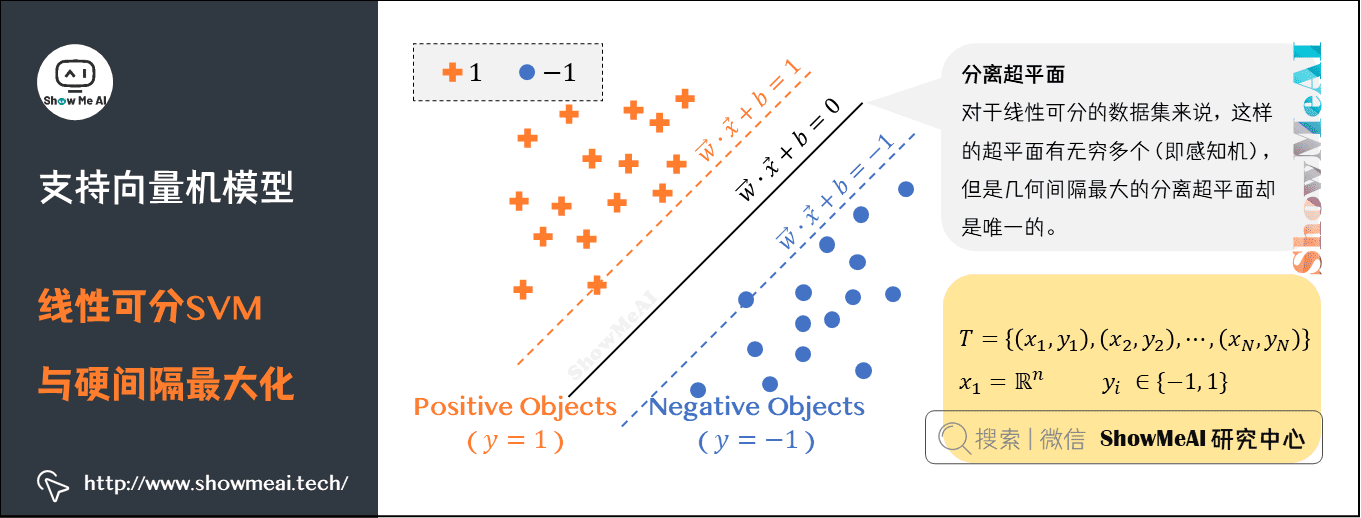### （1）几何间隔

对于给定的数据集 T T 和超平面 w x + b = 0 wx+b=0

• 定义超平面关于样本点 ( x i , y i ) (x_{i}, y_{i}) 的几何间隔为： γ i = y i ( w ∥ w ∥ ⋅ x i + b ∥ w ∥ ) \gamma_{i} = y_i(\frac{w}{\left \| w \right \| } \cdot x_i + \frac{b}{\left \| w \right \| })

• 超平面关于所有样本点的几何间隔的最小值为： γ = min ⁡ i = 1 , 2 … , N γ i \gamma = \min _{i=1,2 \ldots, N} \gamma_{i} ，实际上这个距离就是我们所谓的「支持向量到超平面的距离」。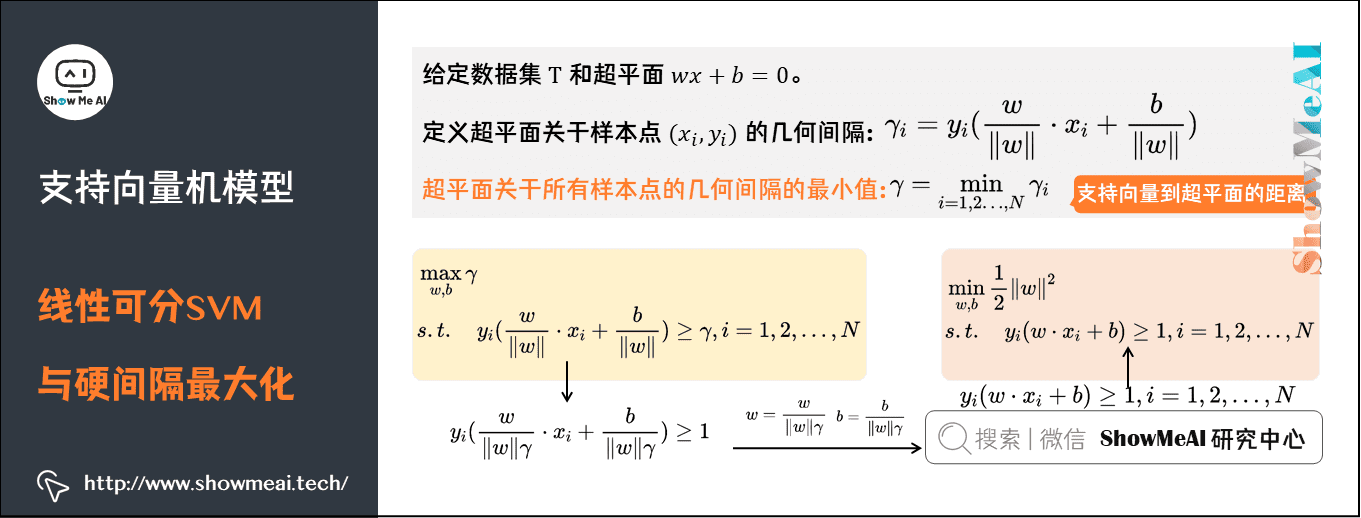① 根据以上定义，SVM模型的「求解最大分割超平面问题」可以表示为以下约束最优化问题

max ⁡ w , b γ s . t . y i ( w ∥ w ∥ ⋅ x i + b ∥ w ∥ ) ≥ γ , i = 1 , 2 , … , N \begin{aligned} & \max _{w,b} \gamma \\ & s.t. \quad y_{i}(\frac{w}{\left \| w \right \|} \cdot x_i+\frac{b}{\left \| w \right \|}) \geq \gamma, i=1,2, \ldots, N\\ \end{aligned}

• 上面公式中s.t是subject to的缩写，也就是限制条件的意思。

② 将约束条件两边同时除以 γ \gamma 得到

y i ( w ∥ w ∥ γ ⋅ x i + b ∥ w ∥ γ ) ≥ 1 y_{i}(\frac{w}{\left \| w \right \| \gamma} \cdot x_i+\frac{b}{\left \| w \right \|\gamma}) \geq 1

③ 因为 ∥ w ∥ \left \| w \right \| γ \gamma 都是标量，所以为了表达式简洁，令： w = w ∥ w ∥ γ w=\frac{w}{\left \| w \right \| \gamma} b = b ∥ w ∥ γ b=\frac{b}{\left \| w \right \| \gamma} 得到

y i ( w ⋅ x i + b ) ≥ 1 , i = 1 , 2 , … , N y_i(w \cdot x_i+b) \geq 1, i=1,2, \ldots, N

④ 又因为最大化 γ \gamma ，等价于最大化 1 ∥ w ∥ \frac{1}{\left \| w \right \|} ，也就等价于最小化 1 2 ∥ w ∥ 2 \frac{1}{2}\left \| w \right \|^{2} ，最终SVM模型的求解最大分割超平面问题又可以表示为以下约束最优化问题

min ⁡ w , b 1 2 ∥ w ∥ 2 s . t . y i ( w ⋅ x i + b ) ≥ 1 , i = 1 , 2 , … , N \begin{aligned} & \min _{w, b} \frac{1}{2}\left \| w \right \|^{2}\\ & s.t. \quad y_{i}(w \cdot x_i+b) \geq 1, i=1,2, \ldots, N \end{aligned}

### （2）对偶算法

求解线性可分支持向量机的最优化问题，我们很多时候会将它转化为对偶问题（dual problem）来求解，也就是应用「拉格朗日对偶性」，通过求解「对偶问题（dual problem）」得到「原始问题（primal problem）」的最优解，即线性可分支持向量机的对偶算法（dual algorithm）。

这样做有一些优点：

• 对偶问题往往更容易求解。

• 引入自然核函数，进而可以推广到非线性分类问题。

① 我们首先构建拉格朗日函数（Lagrange function）。为此，对每一个不等式约束引进拉格朗日乘子（Lagrange multiplier） α i ≥ 0 , i = 1 , 2 , … , N \alpha_i\geq 0,i=1,2,…,N ，定义拉格朗日函数：

L ( w , b , α ) = 1 2 w T w + ∑ i = 1 N α i ( 1 − y i ( w T x i + b ) ) = 1 2 w T w − ∑ i = 1 N α i y i ( w T x i + b ) + ∑ i = 1 N α i \begin{aligned} L(w, b, \alpha)=&\frac{1}{2}w^Tw+\sum_{i=1}^{N}\alpha_i(1-y_i(w^T x_i + b)) \\ =& \frac{1}{2}w^Tw-\sum_{i=1}^{N}\alpha_i y_i(w^T x_i + b) +\sum_{i=1}^{N}\alpha_i \end{aligned}

根据拉格朗日对偶性，原始问题的对偶问题是极大极小问题： max ⁡ α min ⁡ w , b L ( w , b , α ) \max_{\alpha}\min_{w,b}L(w, b, \alpha) 。所以，为了得到对偶问题的解，需要先求 L ( w , b , α ) L(w, b, \alpha) w 、 b w、b 的极小值，再求对 $\alpha$ 的极大值。

② 求 L ( w , b , α ) L(w, b, \alpha) w 、 b w、b 的极小值

将拉格朗日函数 L ( w , b , α ) L(w, b, \alpha) 分别对 w 、 b w、b 求偏导，并令其值为0。

∂ L ∂ w = w – ∑ i = 1 N α i y i x i = 0 ⇒ w = ∑ i = 1 N α i y i x i \frac{\partial L}{\partial w} = w – \sum_{i=1}^{N}\alpha_i y_i x_i =0 \Rightarrow w = \sum_{i=1}^{N}\alpha_i y_i x_i

∂ L b = − ∑ i = 1 N α i y i = 0 ⇒ ∑ i = 1 N α i y i = 0 \frac{\partial L}{b}=-\sum_{i=1}^{N}\alpha_iy_i=0 \Rightarrow \sum_{i=1}^{N}\alpha_iy_i=0

将上式代入拉格朗日函数，即得：

min ⁡ w , b L ( w , b , α ) = 1 2 ( ∑ i = 1 N α i y i x i ) T ∑ i = 1 N α i y i x i − ∑ i = 1 N α i y i ( ( ∑ i = 1 N α i y i x i ) T x i + b ) + ∑ i = 1 N α i = 1 2 ∑ i = 1 N ∑ j = 1 N α i y i α j y j x i T x j − ∑ i = 1 N ∑ j = 1 N α i y i α j y j x j T x i + ∑ i = 1 N α i = − 1 2 ∑ i = 1 N ∑ j = 1 N α i y i α j y j x i T x j + ∑ i = 1 N α i \begin{aligned} \min_{w,b} L(w, b,\alpha)=&\frac{1}{2} (\sum_{i=1}^{N}\alpha_i y_i x_i)^T \sum_{i=1}^{N}\alpha_i y_i x_i-\sum_{i=1}^{N}\alpha_i y_i((\sum_{i=1}^{N}\alpha_i y_i x_i)^T x_i + b) +\sum_{i=1}^{N}\alpha_i \\ =&\frac{1}{2}\sum_{i=1}^{N}\sum_{j=1}^{N}\alpha_i y_i\alpha_j y_jx_i^Tx_j-\sum_{i=1}^{N}\sum_{j=1}^{N}\alpha_i y_i\alpha_j y_jx_j^Tx_i+\sum_{i=1}^{N}\alpha_i \\ =& -\frac{1}{2}\sum_{i=1}^{N}\sum_{j=1}^{N}\alpha_i y_i\alpha_j y_jx_i^Tx_j+\sum_{i=1}^{N}\alpha_i \end{aligned}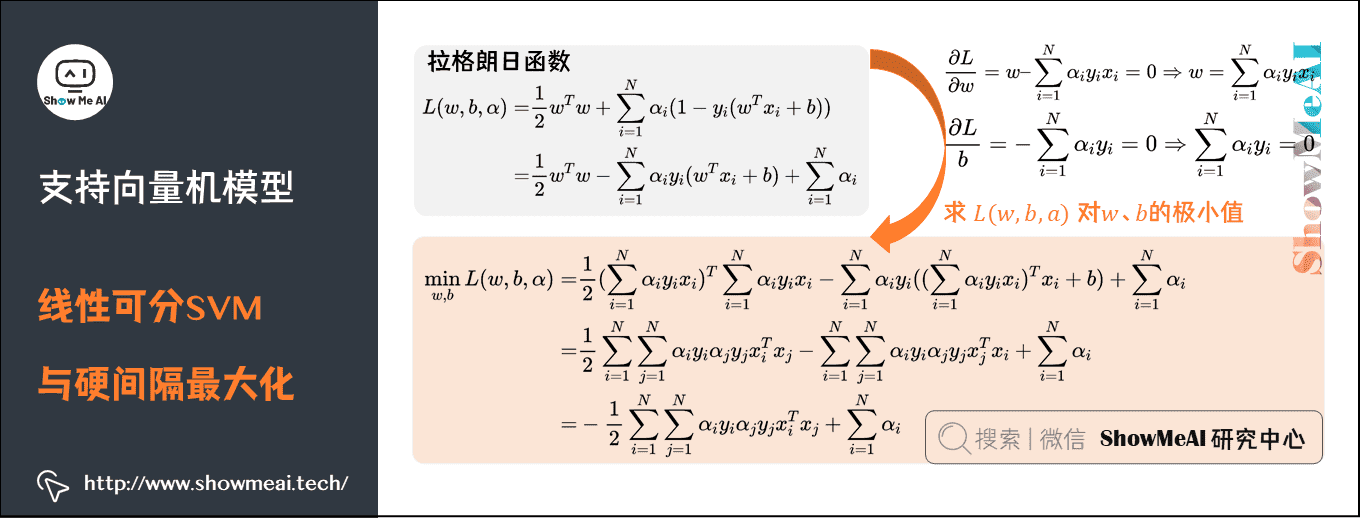③ 求 min ⁡ w , b L ( w , b , α ) \min_{w,b}L(w, b,\alpha) α \alpha 的极大值，即对偶问题

max ⁡ α − 1 2 ∑ i = 1 N ∑ j = 1 N α i y i α j y j x i T x j + ∑ i = 1 N α i s . t . ∑ i = 1 N α i y i = 0 α i ≥ 0 , i = 1 , 2 … N \begin{aligned} & \max_{\alpha} -\frac{1}{2}\sum_{i=1}^{N}\sum_{j=1}^{N}\alpha_i y_i\alpha_j y_jx_i^Tx_j+\sum_{i=1}^{N}\alpha_i \\ & s.t. \quad \sum_{i=1}^{N}\alpha_iy_i=0 \\ & \quad \quad \alpha_i \geq 0,i=1, 2…N \end{aligned}

将式中的目标函数由求极大转换成求极小，就得到下面与之等价的对偶最优化问题：

min ⁡ α 1 2 ∑ i = 1 N ∑ j = 1 N α i y i α j y j x i T x j − ∑ i = 1 N α i s . t . ∑ i = 1 N α i y i = 0 α i ≥ 0 , i = 1 , 2 … N \begin{aligned} & \min_{\alpha} \frac{1}{2}\sum_{i=1}^{N}\sum_{j=1}^{N}\alpha_i y_i\alpha_j y_jx_i^Tx_j-\sum_{i=1}^{N}\alpha_i \\ & s.t. \quad \sum_{i=1}^{N}\alpha_iy_i=0 \\ & \quad \quad \alpha_i \geq 0,i=1, 2…N \end{aligned}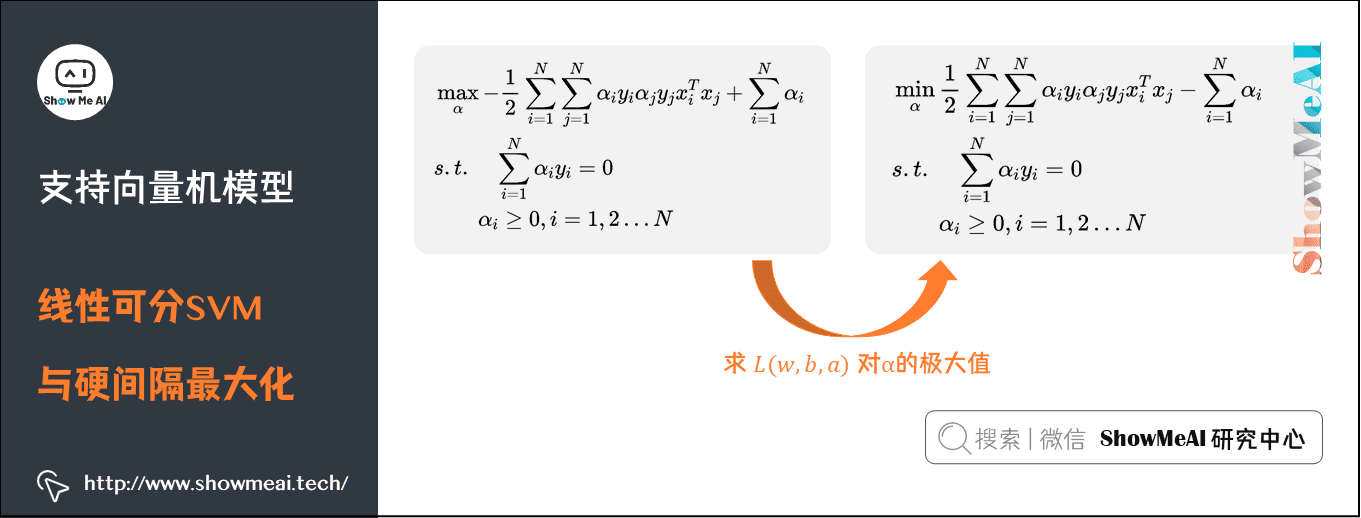**④ 对线性可分训练数据集，假设对偶最优化问题对 α \alpha 的解为 α ∗ = ( α 1 ∗ , α 2 ∗ , … , α N ∗ ) T \alpha^*=(\alpha_1^*,\alpha_2^*,…,\alpha_N^*)^ T ，可以由 α ∗ \alpha^* 求得原始最优化问题的解 w ∗ 、 b ∗ w^*、b^* **。

有下面的定理：设 α ∗ = ( α 1 ∗ , α 2 ∗ , … , α N ∗ ) T \alpha^*=(\alpha_1^*,\alpha_2^*,…,\alpha_N^*)^T 是对偶最优化问题的解，则存在下标 j j ，使得 α j ∗ > 0 \alpha_j^*>0 ，并可按下式求得原始最优化问题的解 w ∗ , b ∗ w^*,b^*

w ∗ = ∑ i = 1 ∗ N α i ∗ y i x i w^*=\sum_{i=1}^*{N} \alpha_i^*y_ix_i

b ∗ = y j − ∑ i = 1 ∗ N α i ∗ y i ( x i ∗ T x j ) b^*=y_j-\sum_{i=1}^*{N} \alpha_i^*y_i(x_i^*Tx_j)

证明：根据定理，KKT条件成立，即得：

∂ L ( w ∗ , b ∗ , α ∗ ) ∂ w = w ∗ − ∑ i = 1 N α i ∗ y i x i = 0 ∂ L ( w ∗ , b ∗ , α ∗ ) ∂ b = − ∑ i = 1 N α i ∗ y i = 0 ∂ L ( w ∗ , b ∗ , α ∗ ) ∂ α = 0 α i ( 1 − y i ( w T x i + b ) ) = 0 α i ≥ 0 , i = 1 , 2 , … , N 1 − y i ( w T x i + b ) ≤ 0 , i = 1 , 2 , … , N \begin{aligned} & \frac{\partial L(w^*,b^*,\alpha ^*)}{\partial w} = w^*-\sum_{i=1}^{N}\alpha_i^* y_i x_i=0 \\ & \frac{\partial L(w^*,b^*,\alpha ^*)}{\partial b} = -\sum_{i=1}^{N}\alpha_i^*y_i=0 \\ & \frac{\partial L(w^*,b^*,\alpha ^*)}{\partial \alpha} = 0 \\ & \alpha_i(1-y_i(w^Tx_i+b)) =0 \\ & \alpha_i \geq 0 , \\ & i=1,2,…,N \\ & 1-y_i(w^Tx_i+b) \leq 0 , i=1,2,…,N \end{aligned}

由此得 w ∗ = ∑ i = 1 N α i ∗ y i x i w^*=\sum_{i=1}^{N} \alpha_i^*y_ix_i

\其中至少有一个 α j ∗ > 0 \alpha_j^*>0 （用反正法，假设 α j ∗ = 0 \alpha_j^*=0 ，由式可知 w ∗ = 0 w^*=0 ，而 w ∗ = 0 w^*=0 不是原始最优化问题的解，产生矛盾），由此对 j j 有： y j ( w ∗ ⋅ x j + b ∗ ) – 1 = 0 y_j(w^*\cdot x_j+b^*) – 1 = 0

将式代入并注意到 y j 2 = 1 y_j^2=1 ，即得： b ∗ = y j – w ∗ x j = y j – ∑ i = 1 N α i ∗ y i ( x i x j ) b^* = y_j – w^*{}x_j = y_j – \sum_{i=1}^{N} \alpha_i^*y_i(x_ix_j)

⑤ 由此定理可知，分离超平面可以写成

∑ i = 1 N α i ∗ y i ( x ⋅ x i ) + b ∗ = 0 \sum_{i=1}^{N} \alpha_i^*y_i(x\cdot x_i) + b^* = 0

⑥ 分类决策函数可以写成

f ( x ) = s i g n ( ∑ i = 1 N α i ∗ y i ( x ⋅ x i ) + b ∗ ) f(x) = sign(\sum_{i=1}^{N} \alpha_i^*y_i(x\cdot x_i) + b^*)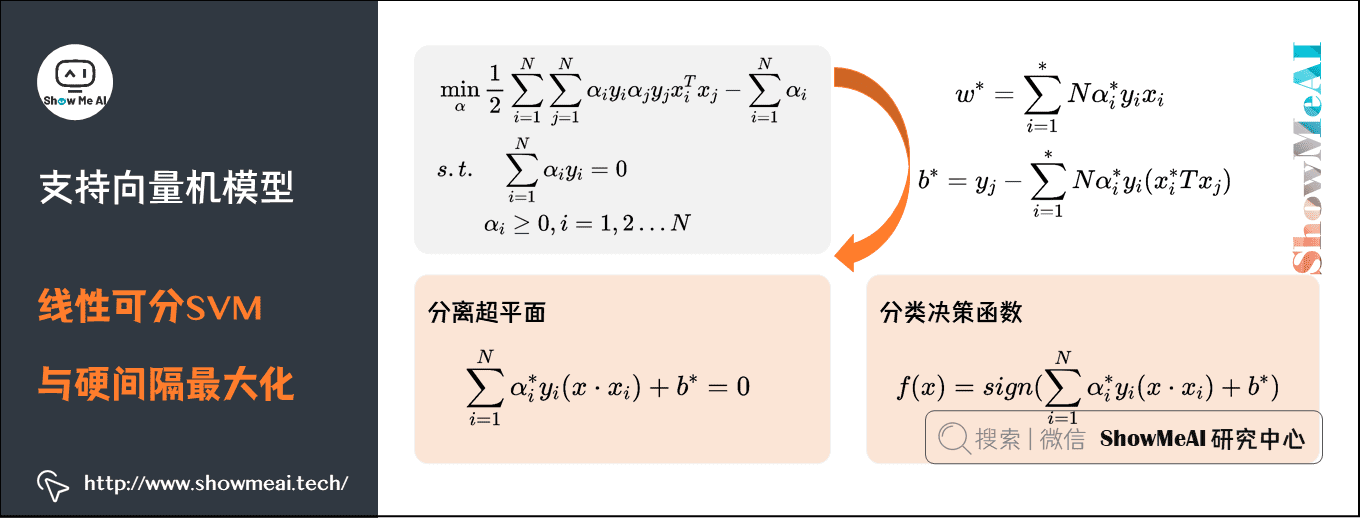也就是说，这里的决策函数只依赖于输入 x x 和训练样本输入的内积。上式亦称作线性可分SVM的对偶形式。

综上所述，对于给定得线性可分训练数据集，可以首先求对偶问题的解 α ∗ \alpha^* ；再利用公式求得原始问题的解 w ∗ , b ∗ w^*,b^* ；从而得到分离超平面及分类决策函数。这种算法称为线性可分支持向量机的对偶学习算法，是线性可分支持向量机学习的基本算法。

## 2）线性SVM与软间隔最大化

我们前面提到的是线性可分的情况，但实际应用中完全线性可分的情况太少见了。如下图就是一个典型的线性不可分的分类图（我们没有办法找到一条直线，把空间划分为2个区域，一个区域只有黑点，一个区域只有白点）。

要对其进行切分，有2种方案：

方案1：用曲线去将其完全分开，即非线性的决策边界，这会和之后谈到的核函数关联方案2：还是使用直线，不过不追求完全可分，会适当包容一些分错的情况，在这个过程中我们会在模型中加入惩罚函数，尽量让分错的点不要太多太离谱。对分错点的惩罚函数就是这个点到其正确位置的距离，如下图所示：图上黄色、蓝色的直线分别为支持向量所在的边界，黑色的线为决策函数，那些绿色的线表示分错的点到其相应的决策面的距离，这样我们可以在原函数上面加上一个惩罚函数，并且带上其限制条件为：

min ⁡ 1 2 ∣ w ∣ 2 + C ∑ i = 1 R ε i , s . t . , y i ( w T x i + b ) ≥ 1 − ε i , ε i ≥ 0 \begin{aligned} & \min \frac{1}{2}|w|^{2}+C \sum_{i=1}^{R} \varepsilon_{i}, \\ & s.t., y_{i}\left(w^{T} x_{i}+b\right) \geq 1-\varepsilon_{i}, \varepsilon_{i} \geq 0 \end{aligned}

上述公式为在线性可分问题的基础上加上的惩罚函数部分，当 x i x_i 在正确一边的时候， ε = 0 \varepsilon = 0 ，R为全部的点的数目，C是一个由用户去指定的系数，表示对分错的点加入多少的惩罚：

• 当C很大的时候，分错的点就会更少，但是过拟合的情况可能会比较严重。
• 当C很小的时候，分错的点可能会很多，不过可能由此得到的模型也会不太正确。

实际我们也会调整和选择合适的C值。

经过这个变换之后，我们可以同样求解一个拉格朗日对偶问题，得到原问题的对偶问题的表达式：

max ⁡ α ∑ i = 1 n α i − 1 2 ∑ i , j = 1 n α i α j y i y j x i T x j  s.t.  , C ≥ α i ≥ 0 , i = 1 , ⋯   , n , ∑ i = 1 n α i y i = 0 \begin{aligned} & \max _{\alpha} \sum_{i=1}^{n} \alpha_{i}-\frac{1}{2} \sum_{i, j=1}^{n} \alpha_{i} \alpha_{j} y_{i} y_{j} x_{i}^{T} x_{j} \\ & \text { s.t. }, C \geq \alpha_{i} \geq 0, i=1, \cdots, n , \sum_{i=1}^{n} \alpha_{i} y_{i}=0 \end{aligned}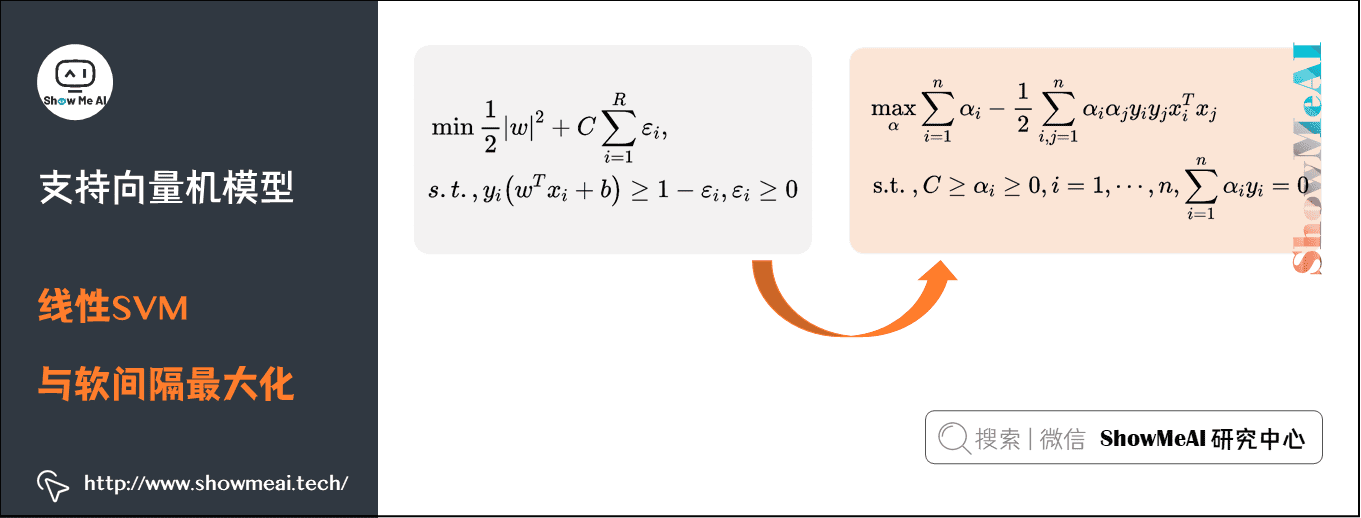在线性不可分情况下得到的对偶问题，不同的地方就是α的范围从[0, +∞)，变为了[0, C]，增加的惩罚 ε \varepsilon 没有为对偶问题增加太多复杂度。

## 3）非线性SVM与核函数

如果我们要处理的分类问题更加复杂，甚至不能像上面一样近似线性可分呢，这种情况下找到的超平面分错的程度太高不太可接受。

对于这样的问题，一种解决方案是将样本从原始空间映射到一个更高维的特征空间，使得样本在这个特征空间内线性可分，然后再运用SVM求解，如下图所示：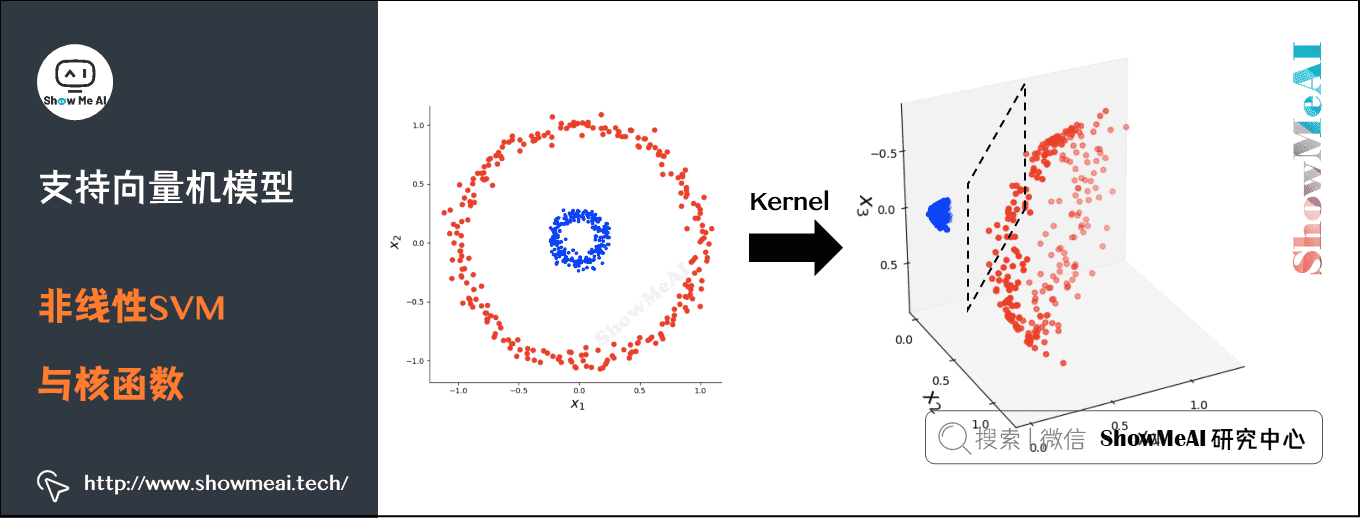比如下图中的典型线性不可分的情况：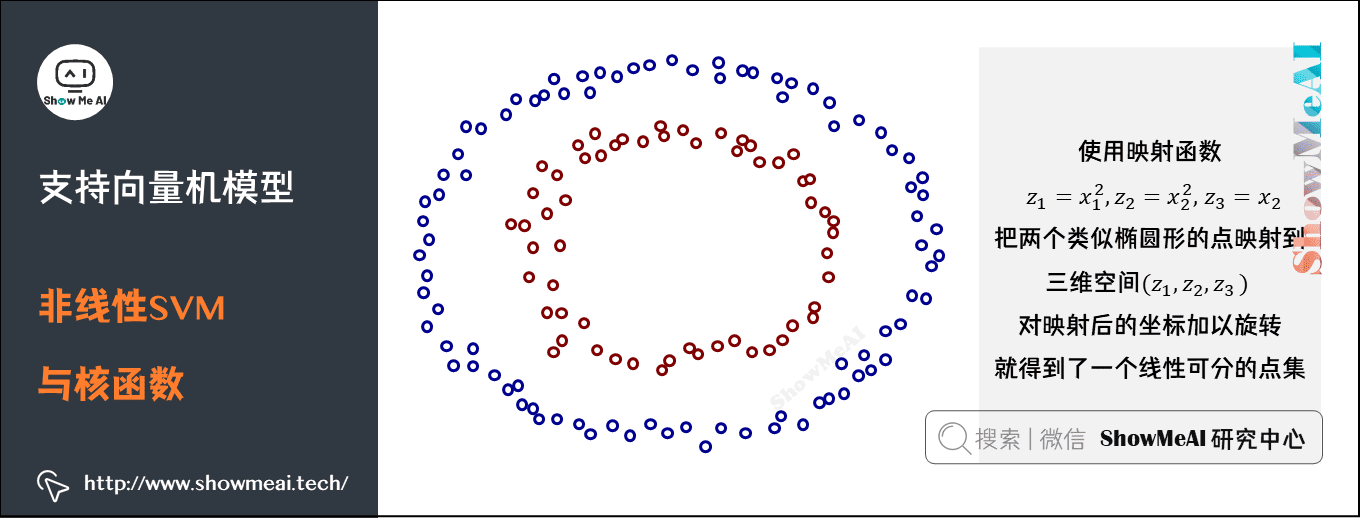当我们使用映射函数 z 1 = x 1 2 , z 2 = x 2 2 , z 3 = x 2 z_{1}=x_{1}^{2}, z_{2}=x_{2}^{2}, z_{3}=x_{2} 把这两个类似于椭圆形的点映射到三维空间 ( z 1 , z 2 , z 3 ) (z_1,z_2,z_3) 后，对映射后的坐标加以旋转之后就可以得到一个线性可分的点集了。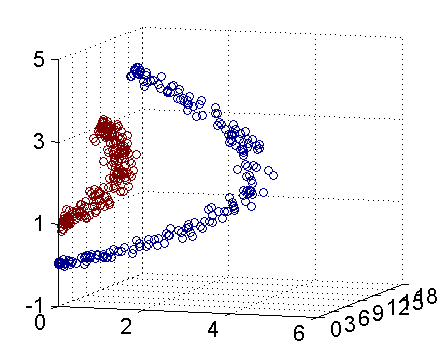我们回忆一下之前得到的对偶问题表达式：

max ⁡ α ∑ i = 1 n α i − 1 2 ∑ i , j = 1 n α i α j y i y j x i T x j s . t . , C ≥ α i ≥ 0 , i = 1 , ⋯   , n , ∑ i = 1 n α i y i = 0 \begin{aligned} & \max _{\alpha} \sum_{i=1}^{n} \alpha_{i}-\frac{1}{2} \sum_{i, j=1}^{n} \alpha_{i} \alpha_{j} y_{i} y_{j} x_{i}^{T} x_{j} \\ & s.t. , C \geq \alpha_{i} \geq 0, i=1, \cdots, n, \sum_{i=1}^{n} \alpha_{i} y_{i}=0 \\ \end{aligned}

做一点小小的改造，令： x i T x j = κ ( x i , x j ) x_{i}^{T} x_{j}=\kappa\left(x_{i}, x_{j}\right) 。这个式子所做的事情就是将线性的空间映射到高维的空间， κ ( x i , x j ) \kappa\left(x_{i}, x_{j}\right) 有很多种，下面是比较典型的两种：

κ ( x i , x j ) = ( x i ⋅ x j + 1 ) d \kappa\left(x_{i}, x_{j}\right)=\left(x_{i} \cdot x_{j}+1\right)^{d}

κ ( x i , x j ) = exp ⁡ ( − ( x i − x j ) 2 2 σ 2 ) \kappa\left(x_{i}, x_{j}\right)= \exp \left(-\frac{\left(x_{i}-x_{j}\right)^{2}}{2\sigma^{2}}\right)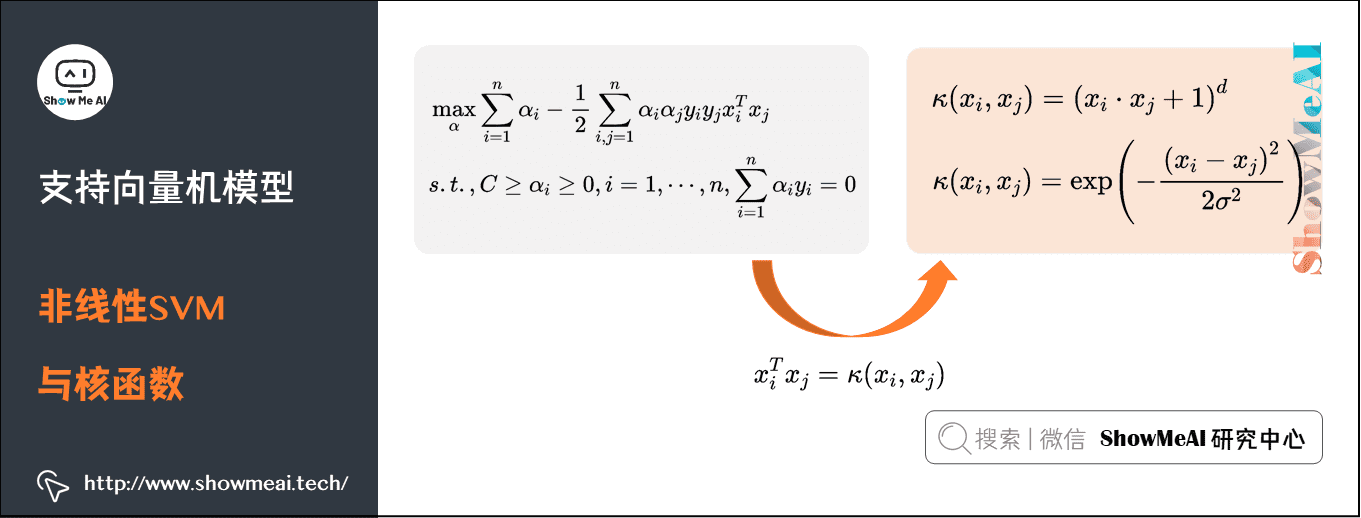上述两个核函数分别为多项式核和高斯核，高斯核甚至是将原始空间映射为无穷维空间，另外核函数有一些比较好的性质，比如说不会比线性条件下增加多少额外的计算量，等等，此处我们不深入。对于一个问题，不同的核函数可能会带来不同的结果，我们需要做一些尝试来支撑选择（关于这个部分，大家可以看最下方的python实现部分）。

# 3.SVM总结

## 1）模型总结

支持向量机（Support vector machines, SVM）是一种二分类模型，它的基本模型是定义在特征空间上的间隔最大的线性分类器，他的学习策略就是间隔最大化，同时该方法可以形式化为一个求解图二次规划。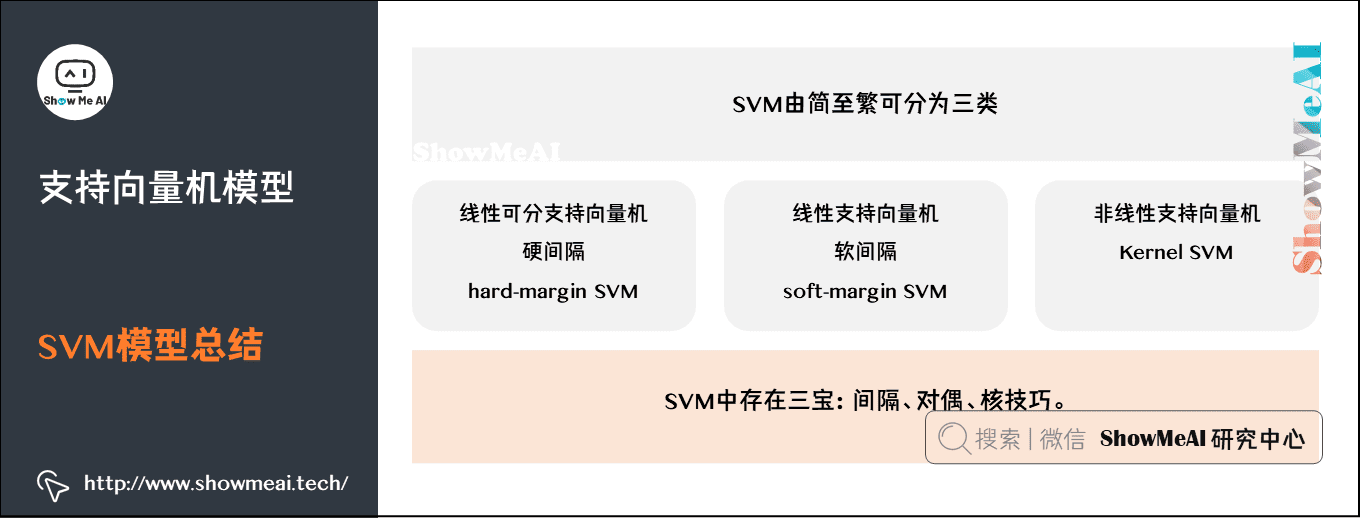SVM由简至繁可分为三类：线性可分支持向量机、硬间隔（hard-margin svm）；线性支持向量机、软间隔（soft-margin svm）；非线性支持向量机、Kernel SVM。

SVM中存在三宝：间隔、对偶、核技巧。

## 2）模型优缺点

### （1）SVM模型优点• SVM是一个凸优化问题，求得的解一定是全局最优而不仅仅是局部最优。

• 不仅适用于线性问题，还适用于非线性问题（借助核技巧）。

• 模型鲁棒性好，决策边界只取决于支持向量而不是全部数据集。

• 中小样本量数据集上效果优异。

• 无需依赖整个数据。

• 泛化能力比较强。

### （2）SVM模型缺点

• 二次规划问题求解将涉及n阶矩阵的计算（其中n为样本的个数），计算量随样本量上涨厉害，因此SVM不适用于超大数据集。

• 原始SVM仅适用于二分类问题。（当然，SVM的推广SVR也适用于回归问题；我们也可以通过one-vs-one，one-vs-rest等思路组合SVM来解决多分类问题）。

• 对非线性问题没有通用解决方案，有时候很难找到一个合适的核函数。

• 对于核函数的高维映射解释力不强，尤其是径向基函数。

• SVM对缺失数据敏感。

更多监督学习的算法模型总结可以查看ShowMeAI的文章AI知识技能速查 | 机器学习-监督学习

# 4.基于Python的SVM代码实践

## 1）算法包说明

我们这里直接借助于python机器学习工具包sklearn来演示SVM的应用，sklearn中对SVM的算法实现都包在sklearn.svm下面，具体见sklearn官方文档，其中SVC类是用来进行进行分类的任务，SVR是用来进行数值回归任务的。

不同的核函数需要指定不同的参数

• 针对线性函数，只需要指定参数C，它表示对不符合最大间距规则的样本的惩罚力度。

• 针对多项式核函数，除了参数C外，还需要指定degree，它表示多项式的阶数。

• 针对高斯核函数，除了参数C外，还需要指定gamma值，这个值对应的是高斯函数公式中 1 2 σ 2 \frac{1}{2\sigma ^2} 的值。

## 2）使用线性核函数

import numpy as np
import matplotlib.pyplot as plt
from sklearn import svm, datasets

X = iris.data[:, :2]  # 只取前两维特征，方便可视化
y = iris.target

svc = svm.SVC(kernel='linear', C=1).fit(X, y)

x_min, x_max = X[:, 0].min() - 1, X[:, 0].max() + 1
y_min, y_max = X[:, 1].min() - 1, X[:, 1].max() + 1
h = (x_max / x_min) / 100
xx, yy = np.meshgrid(np.arange(x_min, x_max, h), np.arange(y_min, y_max, h))

plt.subplot(1, 1, 1)
Z = svc.predict(np.c_[xx.ravel(), yy.ravel()])
Z = Z.reshape(xx.shape)
plt.contourf(xx, yy, Z, cmap=plt.cm.Paired, alpha=0.8)

plt.scatter(X[:, 0], X[:, 1], c=y, cmap=plt.cm.Paired)
plt.xlabel('Sepal length')
plt.ylabel('Sepal width')
plt.xlim(xx.min(), xx.max())
plt.title('SVC with linear kernel')
plt.show()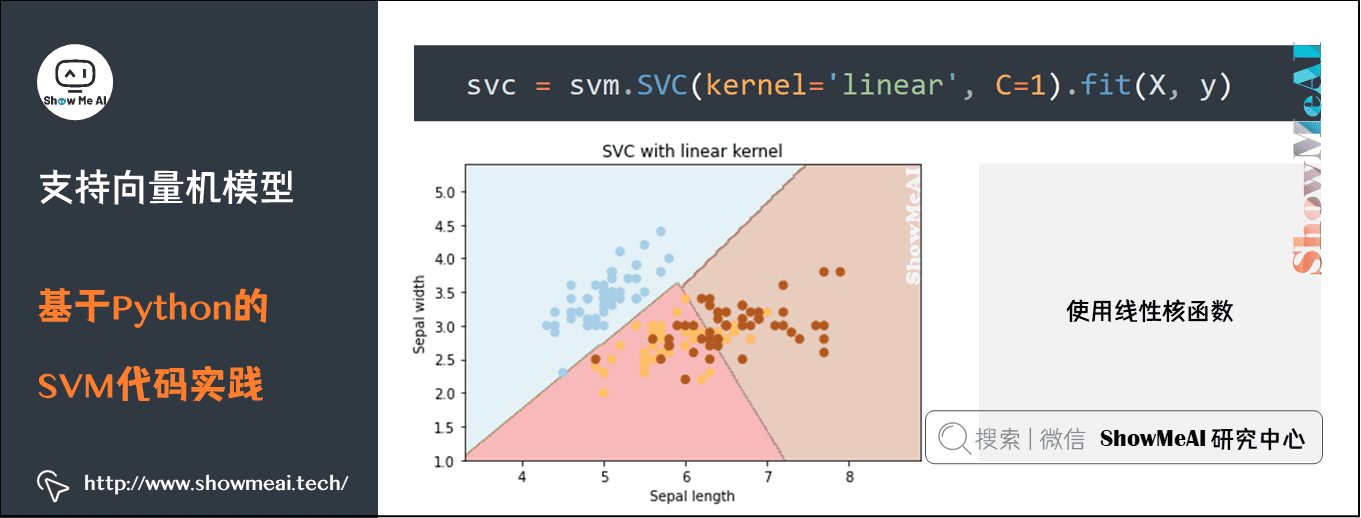## 3）使用多项式核函数

初始化svm对象的代码替换为下面这行

svc = svm.SVC(kernel='poly', degree=3).fit(X, y)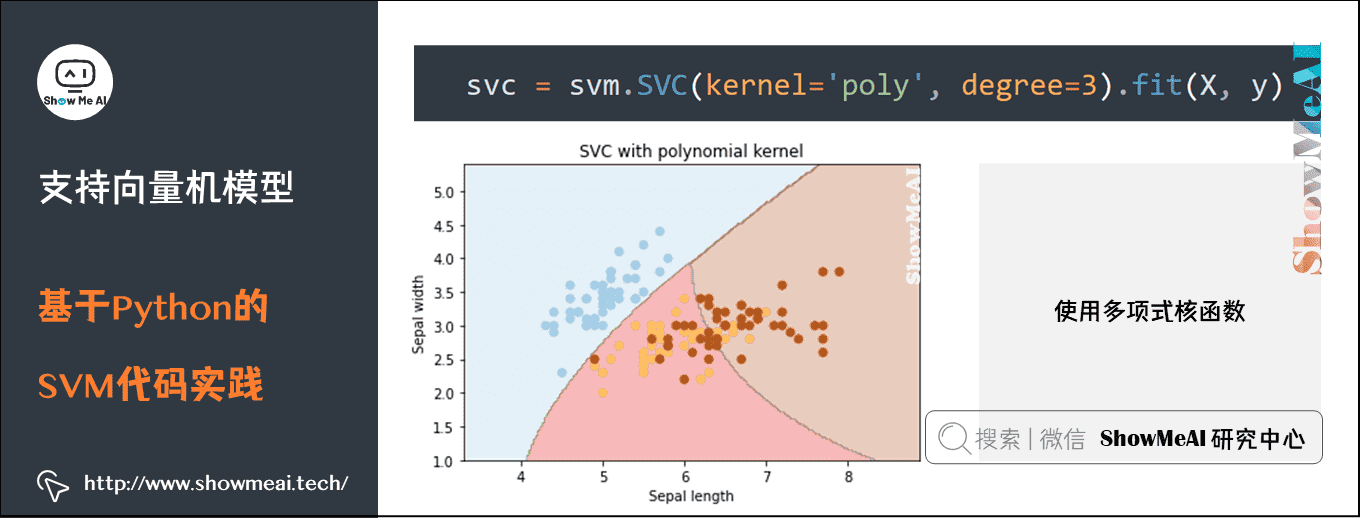## 4）使用rbf核函数（高斯核函数）

初始化svm对象的代码替换为下面这行

svc = svm.SVC(kernel='rbf', C=1).fit(X, y)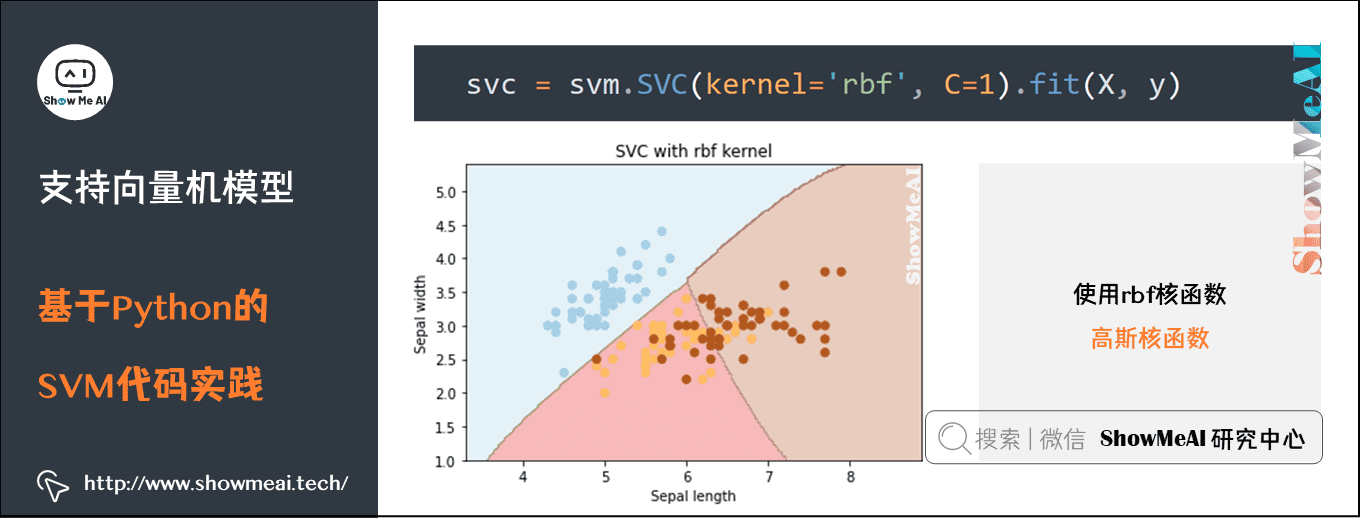gamma 值越大，SVM 就会倾向于越准确的划分每一个训练集里的数据，这会导致泛化误差较大和过拟合问题。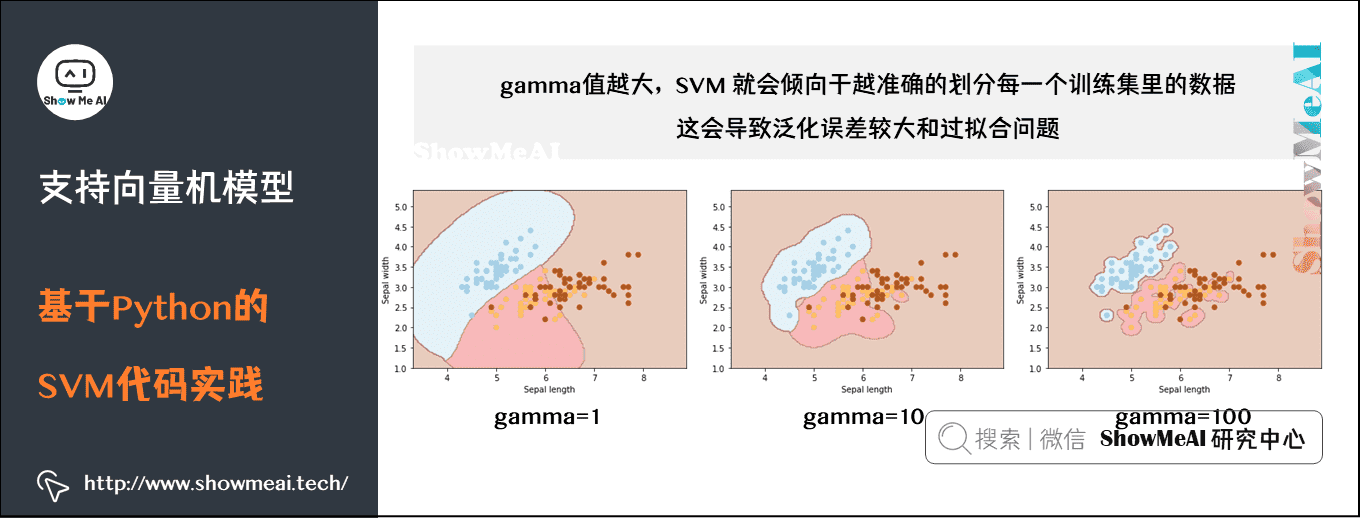C：错误项的惩罚参数C。它还控制平滑决策边界和正确分类训练点之间的权衡。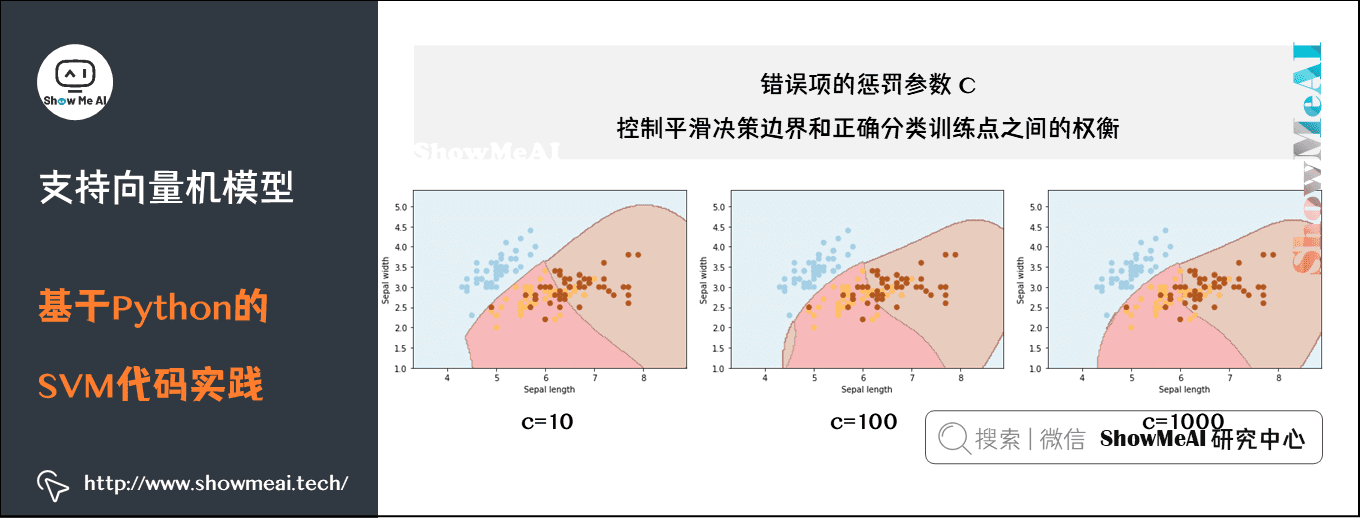# 参考链接

## 视频教程

可以点击 B站 查看视频的【双语字幕】版本

【双语字幕+资料下载】斯坦福CS229 | 机器学习-吴恩达主讲(2018·完整版)

【双语字幕+资料下载】斯坦福CS229 | 机器学习-吴恩达主讲(2018·完整版)

https://www.bilibili.com/video/BV1TT4y127Nf?p=6

## ShowMeAI系列教程推荐展开全文机器学习 人工智能 svm 算法
• 机器学习（六）~支持向量机1. 支持向量机模型2. 线性可分支持向量机2.1 原始问题2.2 对偶问题及求解2.3 线性可分支持向量机算法3. 线性支持向量机3.1 软间隔最大化3.2 对偶问题及求解3.3 线性支持向量机算法4. 非...机器学习 算法 数据挖掘
• 【考试要求】1.理解平面向量数量积的含义及其物理意义；2.了解平面向量的数量积与向量投影的关系；...平面向量数量积的有关概念【考点聚焦】考点一　平面向量数量积的运算【规律方法】　1.数量积公式a·b＝|a||b|...
• 高中数学《平面向量的数量积》说课稿　作为一位无私...　一、说教材　平面向量的数量积是两向量之间的乘法，而平面向量的坐标表示把向量之间的运算转化为数之间的运算。本节内容是在平面向量的坐标表示以及平面向...
• 写在前面初次见面，请多多关照——阿拉丁平面向量基本定理在上一节中我们吐槽了向量的名字，这一节我们就要来考虑向量的好朋友——数形结合。平面内任何向量改如何表示？我们知道在我们熟悉的自然数里1作为一个特殊...
• 我们有很多点，要找一个平面使得每一个点投影到这个法向量上面的数值之最小。 乘积即为投影 Cn都是独立的，我们先看c, c就是下图右边的中心点： 然后对其进行一次正规化：也就是减去其中值，就可以将问题转化...自动驾驶
• 从拉格朗日乘子法、对偶条件核技巧出发 以数学推导的角度来解析SVM的原理机器学习 算法 矩阵
• 第15讲 子空间投影...从图中可以看出，这个距离最近的点p就位于穿过b点并与向量a正交的直线与向量a所在直线的交点上。这就是b在a上的投影。如果我们将向量p视为b的一种近似，则长度e=b-p就是这一近似的误差。...
• 关键是求出平面上的所有点满足的方程，现在知道一个点还有法向量，那么在平面上取一个点用它那个点组成向量，这个向量与法向量的点积为零，就求完了 三点式 共面就是三个向量的混合积为零，然后以减少x，y，z出现...
• 支持向量机，因其英文名为 support vector machine，故一般简称 SVM，通俗来讲，它是一种二类分类 模型，其基本模型定义为特征空间上的间隔最大的线性分类器，其学习策略便是间隔最大化，最终可转化 为一个凸二次...
• 支持向量机（Support vector machine, SVM）是一种二分类模型，是...**SVM 的分割策略是间隔最大化，通过寻求结构化风险最小来提高模型的泛化能力，实现经验风险置信范围的最小化。**SVM 可以转化为求解凸二次规划的算法 python 机器学习
• 向量法是解高中立体几何题的神器。只要能建立空间直角坐标系的题，都可以用向量法来解，而这样的题目可以占到所有立体几何题的 95% 以上。与传统方法相比，向量法的计算量稍微大一些，但它的优点是不需要费脑筋做...
• 说起高中数学，除了函数知识点让大家头疼，就是平面向量了，但是平面向量作为高中数学的一个重点知识。关于很多平面向量的概念及线性运算习题都不会做，那么老师一对一辅导分享关于平面向量的概念及线性运算习题。 ...高中数学
• 2018-01-18 创建人：Ruo_Xiao 邮箱：xclsoftware@163.com 法向量N： 点P： 平面方程：
• 1.敘述三种分类学习方法:LDA，朴素贝叶斯，支持向量机的原理算法，三种分类方法各适合什么样的数据集？ 先说结论：LDA适合于样本固定，参数未知但不固定，是个随机变量，服从一定的分布的数据集。 朴素贝叶斯的...算法 机器学习 朴素贝叶斯算法 python
• ## 多元函数的切向量和法向量

万次阅读 多人点赞 2017-12-13 11:25:33
有一个很有启发性的说法：考虑描述曲面的隐...（F'x，F'y，F'z）是曲线的法向量，（dx,dy,dz）则是曲线的切向量。 1 对曲面而言，求各变量在某一点的偏导数，即为这一点的法向量。 切向量我们假设以x为变...
• 3.支持向量到超平面的距离都是相等的 4.硬间隔最大化：超平面能够完美分类正负例，距离超平面最近的点越远越好。 5.怎么确定超平面？ 6.找到一组最好的w,b固定一个超平面，使这个超平面在能完美区分正负...分类 算法
• I'm looking for a way to convert direction vector (X,Y,Z) into Euler angles (heading, pitch, bank). I know that direction vector by itself is not enough to get the bank angle, so there's also another ...
• 支持向量机SVM——最大间隔分离超平面的计算 如何定义两个集合的最优分隔超平面呢？ 找到集合"边界"上的若干点，以这些点为基础计算超平面的方向，以二维坐标平面为例子wTx+b=0w^Tx+b=0wTx+b=0，当系数wTw^TwT确定...
• 算法简介 支持向量机(SVM)是种二类分类模型...支持向量机学习方法包含构建由简至繁的模型:线性可分支持向量机、线性支持向量机以及非线性支持向量机。简单模型是复杂模型的基础，也是复杂模型的特殊情况。当训练数据线算法 机器学习
• 我们了解了向量和矩阵，以及它们如何在机器学习中提供帮助。让我们从剩下的地方开始讨论，并涵盖机器学习数学第3部分中有关矩阵的一些剩余详细信息。逆矩阵：我们将从该系列的前2部分讨论的问题开始机器学习数学的第...
• 支持向量机（SVM）是一种二分类模型，它的思想感知机很相似，也是在空间中找到一个超平面将正负样本点分开，也可以说SVM是在感知机基础上发展来的。所以在讲完感知机之后马上开始讲支持向量机。 1. 从感知机到...SVM 线性
• 学数值计算还有复变函数了喔，矩阵忘干净了。又看了一遍 蓝棕 的相关的讲解，总结一下。1.向量是什么？从初到末的箭头(物理角度，表示一种运动过程)有序的数字列表(计算机/数学角度)...比如，规定好坐标平面的单位，...
• word2vec原理（一）： CBOW与Skip-Gram模型基础 word2vec原理（二）：基于...1. 词向量基础 1.1One-Hot 编码（独热编码） 1.2 分布式表示：词向量/词嵌入 1.3 词向量可视化 1.4 用词嵌入做迁移学习 2. ...自然语言处理NLP
• 确实因为halcon的文档写的太好了，所以网上关于halcon的博客就很少，点云拟合平面的文档上介绍的比较详细，但是如何从平面模型中读取法向量可确实是难到了，找了三个小时人傻了，然后发现还是一行函数。 首先来说...halcon
• 3.2.1　直线的方向向量平面的法向量 3.2.2　空间线面关系的判定(一) 学习目标 1.掌握空间点、线、面的向量表示. 2.理解直线的方向向量平面的法向量的意义；会用待定系数法求平面的法向量. 3.能用向量法证明直线...
• 本节课的学习，可以培养学生提出猜想、验证猜想、作出数学发现的意识，增强“平面化”“降维”的转化思想，以及发展空间想象能力。 二、教学目标 1、能用向量语言表述直线与直线、直线与平面平面平面的垂直...r语言 算法...